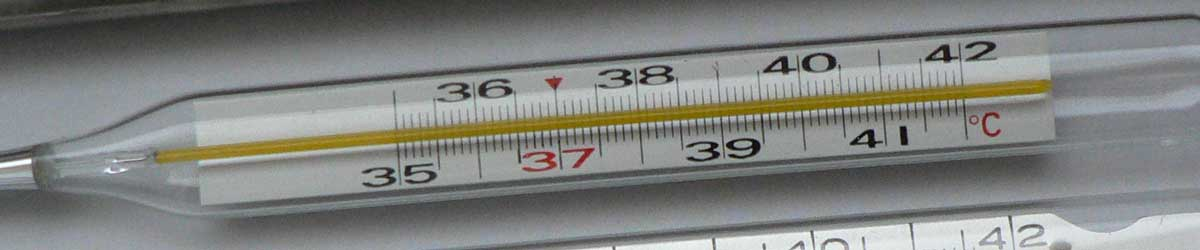Convert Delisle to Kelvins

Enter the temperature in Delisle below to get the value converted to kelvins.

Results in Kelvins:1 °De = 372.483333 K
Do you want to convert kelvins to Delisle?

How to Convert Delisle to KelvinsConvert degrees Delisle to kelvins with this simple formula:

kelvins = 373.15 - [°De] × 2 / 3

Insert the [°De] temperature measurement in the formula and then solve to find the result.

For example, let's convert 50 °De to kelvins:

50 °De = ( 373.15 - 50 × 2 / 3 ) = 339.816667 K

Delisle and kelvins are both units used to measure temperature. Keep reading to learn more about each unit of measure.

Delisle

The Delisle scale is a temperature scale that sets a fixed zero point equal to the temperature of boiling water. The Delisle scale is uses incrementing degrees as the temperature decreases. It runs from 0 degrees at the boiling point of water to 150 degrees at the the freezing point.

Delisle can be abbreviated as De, for example 1 degree Delisle can be written as 1 °De.

Important Temperatures In Degrees Delisle

TemperatureDegrees Delisle
Absolute Zero559.725 °De
Freezing Point of Water150 °De
Boiling Point of Water0 °De

Kelvins

The Kelvin scale is an absolute temperature scale that uses absolute zero as the starting point. The kelvin is defined in terms of the Boltzmann constant, which is a measure of the energy of motion corresponding to one kelvin. 0 kelvins are equal to −273.15 degrees Celsius, which is the point of absolute zero.

The kelvin is the SI base unit for temperature in the metric system. Kelvins can be abbreviated as K, for example 1 kelvin can be written as 1 K.

The kelvin scale is different from other temperature scales in that it is not expressed in degrees, values are expressed in kelvins. When using the Kelvin scale in writing, the "k" is capitalized, however when using the kelvin as a unit the "k" should be lower-case, even though it is named for a person.

Important Temperatures In Kelvins

TemperatureKelvins
Absolute Zero0 K
Freezing Point of Water273.15 K
Triple Point of Water273.16 K
Boiling Point of Water373.15 K

Delisle Measurements and Equivalent Kelvin Conversions

Common Delisle values converted to the equivalent kelvin value
Delisle Kelvins
-50 °De 406.48 K
-40 °De 399.82 K
-30 °De 393.15 K
-20 °De 386.48 K
-10 °De 379.82 K
0 °De 373.15 K
10 °De 366.48 K
20 °De 359.82 K
30 °De 353.15 K
40 °De 346.48 K
50 °De 339.82 K
60 °De 333.15 K
70 °De 326.48 K
80 °De 319.82 K
90 °De 313.15 K
100 °De 306.48 K
110 °De 299.82 K
120 °De 293.15 K
130 °De 286.48 K
140 °De 279.82 K
150 °De 273.15 K
160 °De 266.48 K
170 °De 259.82 K
180 °De 253.15 K
190 °De 246.48 K
200 °De 239.82 K
300 °De 173.15 K
400 °De 106.48 K
500 °De 39.82 K
600 °De -26.85 K
700 °De -93.52 K
800 °De -160.18 K
900 °De -226.85 K
1000 °De -293.52 K

More Delisle Temperature Conversions

Convert to Fahrenheit
1 °De is equal to 210.8 °F
Convert to Celsius
1 °De is equal to 99.333333 °C
Convert to Rankine
1 °De is equal to 670.47 °R
Convert to Newton
1 °De is equal to 32.78 °N